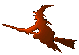Hex  1.0 Hydrogen-electron collision solver
Chebyshev< Tin, Tout > Class Template Reference

Chebyshev approximation. More...

#include <chebyshev.h>

## Public Types

enum  Integration { Integ_Indef, Integ_Low, Integ_High }

## Public Member Functions

Chebyshev ()

Chebyshev (Chebyshev const &cb)

template<class Functor >
Chebyshev (Functor const &f, int n, Tin a, Tin b)
Constructor. More...

Chebyshev (ArrayView< Tout > const &array, Tin a, Tin b)
Constructor from reference to array. More...

template<class Functor >
void generate (Functor const &f, int n, Tin a, Tin b)
Compute the transformation. More...

Tout operator() (Tin const &x) const

Tout clenshaw (Tin const &x, int const &m) const
Evaluate expansion. More...

int tail (double eps) const
Get significant number of coefficients. More...

Tout approx (double x, double eps, int *n=0) const
Evaluate expansion. More...

Chebyshev integrate (Integration itype=Integ_Indef) const
Get expansion of integral of the approximated function. More...

std::string str () const
Convert to string. More...

NumberArray< Tout > const & coeffs () const
Return reference to the coefficient array. More...

template<>
void generate (Functor const &f, int n, double a, double b)

template<>
void generate (Functor const &f, int n, double a, double b)

## Static Public Member Functions

static Tin root (int N, int k, Tin x1=0., Tin x2=1.)
Chebyshev node in interval. More...

static double node (int k, int N)
Chebyshev node from (0,1). More...

## Detailed Description

### template<typename Tin, typename Tout> class Chebyshev< Tin, Tout >

This class manages a Chebyshev approximation

$F(x) = \frac{c_0}{2} + \sum_{k=1}^N c_k T_k(x)$

of a function F with signature

* Tout F (Tin x)
*

## Member Enumeration Documentation

template<typename Tin , typename Tout >

Integration types.

Enumerator
Integ_Indef
Integ_Low
Integ_High

## Constructor & Destructor Documentation

template<typename Tin , typename Tout >
 Chebyshev< Tin, Tout >::Chebyshev ( )
inline
template<typename Tin , typename Tout >
 Chebyshev< Tin, Tout >::Chebyshev ( Chebyshev< Tin, Tout > const & cb )
inline
template<typename Tin , typename Tout >
template<class Functor >
 Chebyshev< Tin, Tout >::Chebyshev ( Functor const & f, int n, Tin a, Tin b )
inline
Parameters
 f Function to approximate. n How many Chebyshev nodes to use. a Left boundary of the approximation interval. b Right boundary of the approximation interval.
template<typename Tin , typename Tout >
 Chebyshev< Tin, Tout >::Chebyshev ( ArrayView< Tout > const & array, Tin a, Tin b )
inline
Parameters
 array Array of precomputed Chebyshev coefficients. a Left boundary of the approximation interval. b Right boundary of the approximation interval.

## Member Function Documentation

template<typename Tin , typename Tout >
 Tout Chebyshev< Tin, Tout >::approx ( double x, double eps, int * n = 0 ) const
inline

Return approximated value where the terms of magnitude less than 'eps' are discarded.

template<typename Tin , typename Tout >
 Tout Chebyshev< Tin, Tout >::clenshaw ( Tin const & x, int const & m ) const
inline

Use Clenshaw recurrence formula for evaluation of 'm' terms. The formula has the advantage of not evaluating goniometric funtions.

template<typename Tin , typename Tout >
 NumberArray const& Chebyshev< Tin, Tout >::coeffs ( ) const
inline
template<typename Tin , typename Tout >
template<class Functor >
 void Chebyshev< Tin, Tout >::generate ( Functor const & f, int n, Tin a, Tin b )

Generate and store the Chebyshev coefficients. After evaluation of the approximated function in the Chebyshev nodes, the computation of Chebyshev expansion coefficients is done using the fast Fourier transform provided by FFTW.

Parameters
 f Function of the signature Tout(*)(Tin) to be approximated. n Number of the coefficients to compute. a Left boundary of the approximation inerval. b Right boundary of the approximation inerval.
template<>
 void Chebyshev< double, double >::generate ( Functor const & f, int n, double a, double b )

Uses the function "fftw_plan_r2r_1d" for real data.

template<>
 void Chebyshev< double, Complex >::generate ( Functor const & f, int n, double a, double b )

Uses the function "fftw_plan_dft_1d" for complex data. Slower than the real "generate".

template<typename Tin , typename Tout >
 Chebyshev Chebyshev< Tin, Tout >::integrate ( Integration itype = Integ_Indef ) const
inline

Return Chebyshev aproximation of the function primitive to the stored Chebyshev approximation.

Parameters
 itype Whether to return general indefinite integral expansion $\int_a^x f(x) \mathrm{d}x \ ,$ (corresponds to "indef") or definite low $\int_0^x f(x) \mathrm{d}x \ ,$ (corresponds to "def_low") or definite high $\int_x^\infty f(x) \mathrm{d}x \ .$
template<typename Tin , typename Tout >
 static double Chebyshev< Tin, Tout >::node ( int k, int N )
inlinestatic

Get k-th root of the N-order Chebyshev polynomial.

template<typename Tin , typename Tout >
 Tout Chebyshev< Tin, Tout >::operator() ( Tin const & x ) const
inline

Return full approximation value.

template<typename Tin , typename Tout >
 static Tin Chebyshev< Tin, Tout >::root ( int N, int k, Tin x1 = 0., Tin x2 = 1. )
inlinestatic

Get Chebyshev root in the interval (x1,x2).

Parameters
 N Order of the polynomial. k index of the root. x1 Left bound of the interval. x2 Right bound of the interval.
template<typename Tin , typename Tout >
 std::string Chebyshev< Tin, Tout >::str ( ) const
inline

Write out the coefficients to std::string.

template<typename Tin , typename Tout >
 int Chebyshev< Tin, Tout >::tail ( double eps ) const
inline

Get index of the first Chebyshev approximation coefficient that is smaller than 'eps' times the sum of fabs(C[k]) truncated after this term. If no such term exists, the total count of terms is returned.

Note
The Chebyshev polynomial corresponding to the last considered term can be negligible near some evaluation point 'x'. In that case, its contribution might be shadowed by the contribution of the following polynomial. So, the evaluated result can have worse precision than the requested 'eps'. Nevertheless, the more polynomials get involved, the less is this fact problematic.

The documentation for this class was generated from the following file: